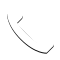Select Page

In this post, I will share with you more about mortgages specifically Adjustable Rate Mortgages (ARM). I will share on how ARM helps to solve problems that are caused by Fixed Rate Mortgages (FRM) that I mentioned in my previous post. I will also show you on the calculation involved in determining your monthly mortgage payment if you choose an ARM. Lastly, you will understand the risk involved in taking an ARM for your financing.

### Problems That Occurs With FRM

##### Interest Rate Risk

Banks often land up in a situation of maturity mismatch. This is because they are liable to return any savings that are saved by savers which is a very short term. However, with a mortgage loan of 20 years, it is a long-term asset. Thus, if FRM interest rate always stays the same but deposit interest rate rises, the amount of revenue will decrease for the bank.

##### Affordability

Imagine if the interest rates are currently very high, you may find the cost to be very high as this same cost will be throughout the whole loan period. However, you cannot change the interest rate payable in future as it is an FRM.

### How Inflation Affect FRMs

Interest rate charged by the bank is: i = r + p + f

• r = riskless interest rate
• p = risk premium
• f = inflation premium
• i: nominal interest rate
• i-f: real interest rate

Scenario: Suppose you take out an FRM for \$60,000 for 30 years with a real rate 4% p.a.

Over time, the bank will lose out because the real value will decrease with inflation.

### How Does ARM Help To Solve Issues?

ARM is designed to solve interest rate problem. Banks are able to adjust contract interest rate periodically to reflect market interest rates. Which means that your interest rate for the loan is not fixed but adjustable. This helps lenders to shift some interest rate risk to the borrowers. If interest rate increases you will also have to bear the risk involved. Lenders often entice you by giving an initial rate that is lower than FRM to secure an ARM loan with you.

### Calculations For ARM

##### Variables
• Teaser(starting) rate for the first period
• Index rate (SIBOR, SOR, 5yr Treasury Bond)
• Margin or spread (additional fixed %)
• Adjustment period of index rate (1yr, 2yr, 3yr)
• Caps and floors (Limit the magnitude of the adjustment)
• Periodic cap limits the amount of increase and decrease of the pay rate for each period (limit per period basis, ±2% per year)
• Lifetime cap (maximum increase in interest rate from starting rate, ±6% lifetime)
##### Contract & Pay Rate
• Contract rate: i = index + margin (SIBOR + 2%)
• Pay rate: The rate of actual payment to the bank (may be different from contract rate due to cap)
• First period pay rate = teaser/starting rate

Example to determine pay rate:

• Teaser Rate = 4.5%
• Periodic cap/floor = ±2%
• Lifetime cap/floor = ±5%
• Index rates are assumed to be as such
 Year Index Rate Margin Contract Rate △% from previous period △% from initial rate Pay Rate 1 4% 2% 6% na na 4.5% 2 5% 2% 7% 2.5% 2.5% 6.5% 3 7% 2% 9% 2.5% 4.5% 8.5% 4 8% 2% 10% 1.5% 5.5% 9.5%

For year 2, even if contract rate is 7% the maximum increase due to the cap is 2% thus pay rate can only be 6.5%. For year 4, even though increase is only 1.5%, however, the lifetime cap is 5% as such pay rate would increase to 9.5% only.

##### ARM Example
• Loan amount = \$150,000
• Index = 1 year TB yield (4% now)
• Adjustment period = 1 year
• Margin = 200BP (1 basis point = 0.01%)
• Maturity = 30 years
• Caps and Floors = ±2% per year, ±6% lifetime
• Monthly payment
• Teaser/starting rate = 4.5%
 Year Initial Balance Index Rate Margin Contract Rate % Pay Rate % Months left = N Monthly Payment 1 \$150,000 4% 2% 6% 4.5% 360 760 2 \$147,574 4% 2% 6% 6% 348 895 3 \$145,499 7% 2% 9% 8% 336 1086 4 \$144,048 5% 2% 7% 7% 324 991

By using a financial calculator, the IRR, the cost of borrowing will amount to 6.28%. Usually, we will give an assumption of the index rate to calculate the effective borrowing cost.

### Risk Of ARMs

• Borrowers may have a hard time understanding how it works
• Lenders may not be able to set the appropriate teaser rate
• As ARM interest rates fluctuate periodically, you may face a greater risk of default if index rate change drastically

## Get In Touch

7 + 4 =Click To Call